## ACT test prepthat actually works

### Vectors: Direction and Magnitude

(5.9.2019)

Brush up on your right triangle skills to handle the most difficult of vector items! #newACTcontent #math #ACT #36U

Step 1: Use the Pythagorean theorem to find the magnitude of the vector.

The vector’s magnitude is approximately 3.5 meters per second.

Step 2: Use a trig ratio to find the direction of the vector.

In this case, we set up the tangent ratio to find the missing angle. The vector’s direction is approximately 31 degrees south of east.

### ACT Math Tip – Period and Amplitude

(2.11.2019)

Recognize period and amplitude from trig graphs—even if you haven’t had Advanced Algebra! #mathmonday #math #ACT #ACTPrep #36U

period = distance for function to complete one full cycle

amplitude = difference between maximum and minimum function values

### November 2018

#### Solution PageWe at 36U want to thank the many students and teachers who participated in our November 2018 Winter Challenge. We hope you enjoyed working the item and learned a new skill or two along the way!

For those who missed it, here was the challenge problem:

The Problem

In a fountain, there are 1,000 quarters, 2,000 dimes, 2,000 nickels, and 5,000 pennies. A student randomly draws a coin from the fountain each day for 3 years, records its value, and tosses it back into the fountain.

Let x equal the value of a coin drawn randomly from the fountain. If all coins are equally likely to be drawn, regardless of coin size, what is the expected value (long-run average) of x?

A. 1¢

B. 4¢

C. 6¢

D. 8¢

E. 10¢

The Solution

The expected value, or long-run average, of coins drawn randomly from the fountain is 6 cents.

Almost every submission was correct. Great work!

Here’s how we worked the item:

Step 1: Find the probability of each outcome.

The first step in solving expected value items is to find the probability of each outcome. Often, the ACT will provide those probabilities in a probability distribution table.

Finding the probabilities will allow us to weight each outcome in Step 2.

Here’s the probability of each outcome:

P(penny) = 5,000 pennies/10,000 total coins = 1/2

P(nickel) = 2,000 nickels/10,000 total coins = 1/5

P(dime) = 2,000 dimes/10,000 total coins = 1/5

P(quarter) = 1,000 quarters/10,000 total coins = 1/10

Step 2: Weight each outcome.

Now that you know the probability of each outcome, weight each outcome value by multiplying by its probability.

One-half of the time, the student draws a penny that is worth 1 cent.

1/2 •  1¢ –> .5¢

One-fifth of the time, the student draws a nickel that is worth 5 cents.

1/5 •  5¢ –> 1¢

One-fifth of the time, the student draws a dime that is worth 10 cents.

1/5 •  10¢ –> 2¢

One-tenth of the time, the student draws a quarter that is worth 25 cents.

1/10 •  25¢ –> 2.5¢

The probabilities add to 1. When we multiplied the probabilites by the values, we essentially broke down a single draw from the fountain into all the possible outcomes, weighted, of course.

Now it’s time to add those values to see what an average draw from the fountain would give.

Expected value = .5¢ + 1¢ + 1¢ + 2.5¢

Expected value = 6¢

The expected value, or long-run average, of a coin drawn randomly from the fountain is 6¢.

Impressive solution!

One of our favorite problem solving approaches came via Facebook. Check it out.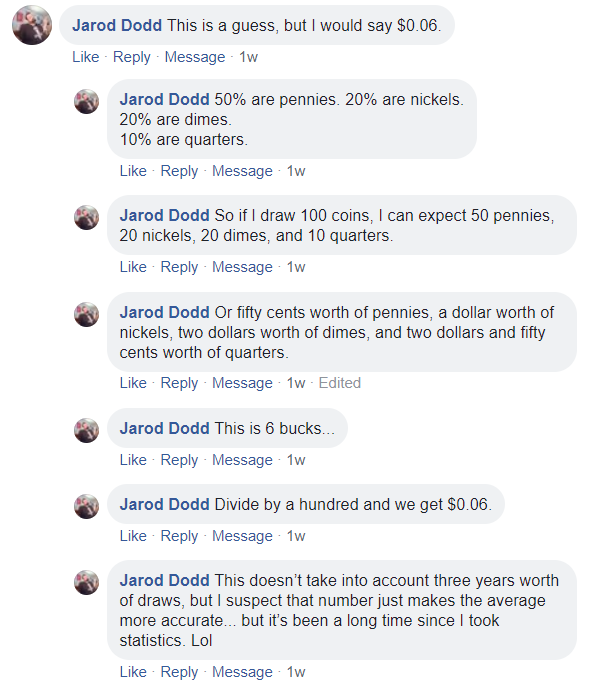The Winner

Congratulations to Bailey S., our November 2018 Expected ValueChallenge winner! Her solution was simple, precise, and just as important, correct. Here’s what she had to say: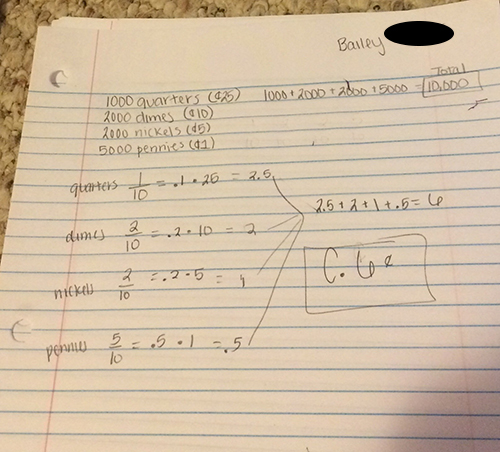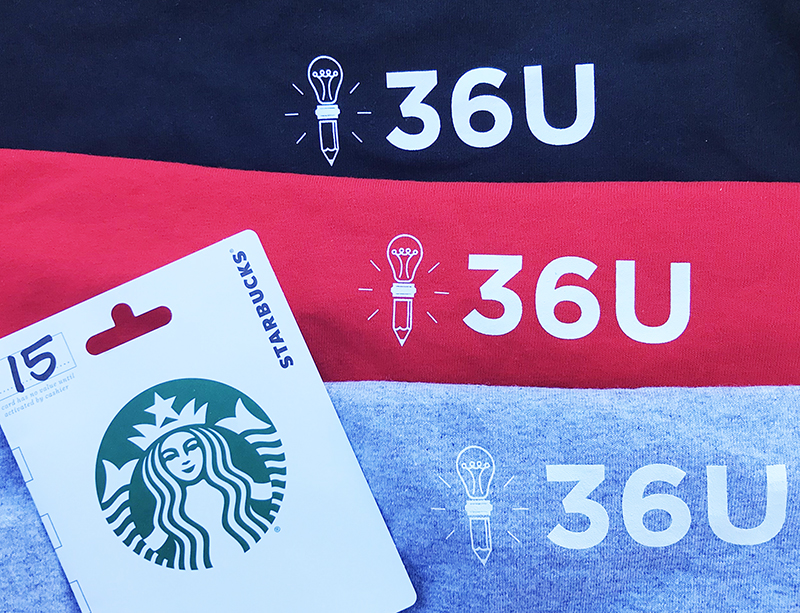The Winnings!

Bailey won the 36U Winter Care Package:

36U Tee (Long-sleeve)

### Expected Value Challenge Item Giveaway

#### November 2018

Statistics and probability are a new focus for the ACT.  We at 36U have come up with a fun challenge to help you prepare for this new focus area–AND to give you a chance to win prizes!

In a fountain, there are 1,000 quarters, 2,000 dimes, 2,000 nickels, and 5,000 pennies. A student randomly draws a coin from the fountain each day for 3 years, records its value, and tosses it back into the fountain.

Let x equal the value of a coin drawn randomly from the fountain. If all coins are equally likely to be drawn, regardless of coin size, what is the expected value (long-run average) of x?

A. 1¢

B. 4¢

C. 6¢

D. 8¢

E. 10¢

How Do I Enter the Contest?

Take a pic of your solution, with your work included, and post as a reply to any of our Expected Value Fountain Challenge social media posts OR email to support@36university.com. Submissions must be posted by midnight eastern time on November 15th. Impress us with your easily-understood, clear solution!What Will I Win?

The winner will receive the 36U Winter Care Package:

36U Tee (Long-sleeve)

How Will 36U Choose a Winner?

At 36U, we value simple, precise solutions. We will draw a winner on November 16th from among entries with correct answers and easy-to-understand explanations. The winner’s submission will be included in  our solution post.

New ACT Math Test Content Part 1: The Factorial

Combinatorics has been added to the ACT math test—and 36U is here to get you ready!

Don’t worry. Combinatorics may sound intimidating, but it’s really about fancy, advanced counting techniques that can be a lot of fun and save lots of time. Let’s get right to it.Factorials

First, let’s work on a possibly new concept with some new notation—the factorial.

6 factorial, written 6!, means 6 • 5 • 4 • 3 • 2 • 1.

And, again:

5! = 5 • 4 • 3 • 2 • 1

To simplify, 5! = 5 • 4 • 3 • 2 • 1 = 120.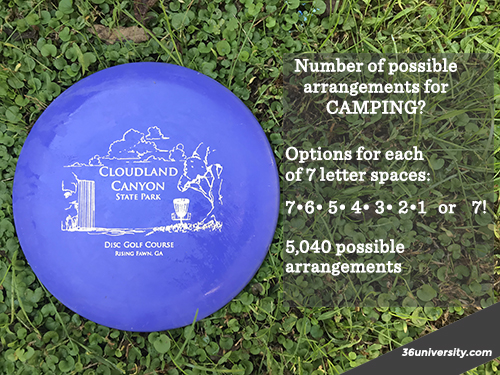Let’s put the factorial to work…

Example 1:  In how many different ways can you arrange the letters of the word OVERCAST?

____  ____  ____  ____  ____  ____  ____  ____

You have 8 options for the first letter, 7 options for the 2nd, 6 options for the 3rd, and so on.

Mathematically, that looks like this:

_8 options  _7 options  _6 options  _5 options  _4 options  _3 options  _2 options  _1 option

or

8! –>  40,320

There are 40,320 different ways the letters of OVERCAST can be arranged.

Easy so far. Let’s move on…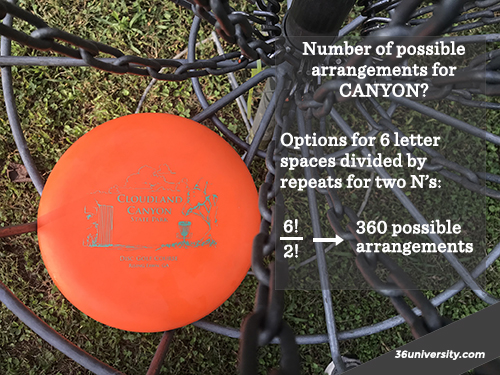Example 2: In how many different ways can you arrange the letters of the word CANYON?

This is a slightly trickier item because CANYON has 2 N’s!  Fortunately, if you understood our first example, this isn’t much more difficult.

Step 1: There are six different spots to place the letters in the word CANYON:

_6 options  _5 options  _4 options  _3 options  _2 options  _1 option     =  6!

There are 6! or 720 different ways of arranging C-A-N-Y-O-N, but…You have to account for repeated options because there are 2 Ns. Here’s how:

Take your total number of possible arrangements (6!) and divide by 2! to account for N appearing twice.

6!/2! –>  (6 • 5 • 4 • 3 • 2 • 1)/(2 • 1)

–>  360

There are 360 different ways the letters of the word CANYON can be arranged!

That’s your introduction to new counting techniques (combinatorics) that are being tested on the ACT.

Take time to brush up on your combination and permutations, too. For more instruction and practice on these topics, check out our online program.

-Dr. Kendal Shipley, 36U

10/13/17

THE Problem I Missed on the ACT Math Test

The original title of this post was supposed to be “The Math Problem I Had to Skip and Come Back To,” but it didn’t work out that way. I formulated the first title after I had to skip THE problem the first time.

I ended up missing the problem!

Recently, ACT Inc put out a new “Official ACT Prep Guide” and, as I always do, I sat down to take the tests (timed, of course) and to categorize the new items. This time, I set the timer and began working.

I was halfway through at the 19-minute mark and had no troubles. I noticed the problems were taking me a little more time than I wanted, but I only had a phone calculator and was only willing to use a small notepad to write something down if absolutely necessary.

No problems so far.

And then I hit #51: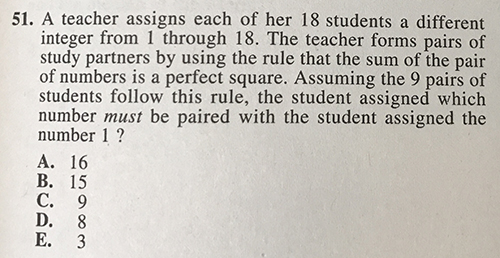(This item is owned by ACT Inc and printed in the first practice test in The Official ACT Practice Guide 2016-2017.)

I made a mental list of all possible perfect square sums from students 1 through 18: 4, 9, 16, 25.  I started working and realized I couldn’t see the “quick way.”

Yes, almost all ACT Math items have a “quick way.”  So, contrary to what I think most students should do at this point (which is to give it their best guess and move on), I skipped it.

I made my way through the rest of the test. The dumb “how many quarters are there?” problem. The Venn diagram problem. The Law of Sines. A probability distribution (That’s new!). And I headed back to #51…

I had about 4 minutes left at this point and decided to continue with the the brute force approach (another critical error?).

I eliminated choices A and C, because 1 + 16 and 1 + 9 don’t give perfect squares.

A little more deduction told me 17 had to be be paired with 8 to give the perfect square 25. Student 17 had to be paired with another student to give a perfect square. There wasn’t a student who could pair with 17 to reach the perfect square 36 (17 + 18 = 35). There was no way to pair student 17 with another student and get a number less than 17, so the only option was to add to 25. That made student 17 pair with student 8.

I was left with choices B and E.

I knew that 1 had to be paired with 3 or 15 (I had known that since the first pass through.). I scribbled. I paired. I scribbled. I paired.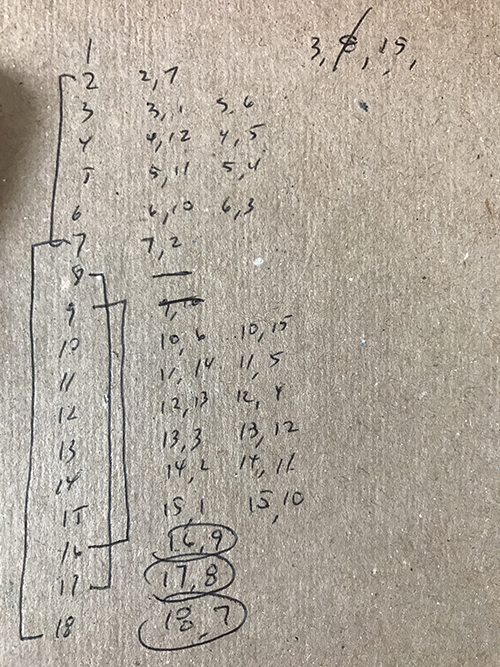And I still didn’t get it. I was running out of time! I chose…3.

Wrong choice!

What Did I Do Wrong?

I’m not really sure where I went wrong. Is there a key that unlocks this problem quickly? I don’t know.

Eventually, I did figure that 1 had to be paired with 15, and this is how I did it…

Pair student 18 with student 7, which is the only option for reaching a perfect square. Likewise, student 17 has to be paired with student 8. Student 16 has to be paired with student 9. Of the nine pairs, we have 3 pairs figured quickly!

Student 15 can be paired with student 10 (sum of 25) or student 1 (sum of 16).

Student 14 can be paired with student 11 (sum of 25) or student 2 (sum of 16).

Student 13 can be paired with student 12 (sum of 25) or student 3 (sum of 16).

Student 12 can be paired with student 13 (sum of 25) or student 4 (sum of 16).

Student 11 can be paired with student 14 (sum of 25) or student 5 (sum of 16).

Student 10 can be paired with student 15 (sum of 25) or student 6 (sum of 16).

Student 9 is already paired with student 16 (see above).

Student 8 is already paired with student 17 (see above).

Student 7 is already paired with student 18 (see above).

Student 6 can be paired with student 10 (sum of 16) or student 3 (sum of 9).

Student 5 can be paired with student 11 (sum of 16) or student 4 (sum of 9).

Student 4 can be paired with student 12 (sum of 16) or student 5 (sum of 9).

Student 3 can be paired with student 13 (sum of 16) or student 6 (sum of 9) or student 1 (sum of 4).

Student 2 can be paired with student 14 (sum of 16), not student 7 (already paired with student 18), not student 2, because he is student 2. We have our fourth pair! Student 2 is paired with student 14!

Student 1 can be paired with student 15 (sum of 16) not student 8 (already paired with student 17) or student 3 (sum of 4).

Step 2: Student 2 is the key.

Student 2 is paired with student 14. From there, work backwards from student to student eliminating possible choices until student 1’s pair is identified.

Go to the Student 14 line. Student 14 is paired with student 2, so that leaves student 11.

Student 11 can’t be paired with student 14. Student 11 is paired with student 5.

Student 5 is paired with student 11, so that leaves student 4.

Student 4 must be paired with student 12.

Student 12 is paired with 4. That leaves student 13.

Student 13 is paired with student 3. The leaves students 1 and 6.

Student 6 is paired with student 10, because student 3 is paired with student 3.

Student 10 is paired with student 6. That leaves student 15.

Student 15 must be paired with student 1!!!

Is there a better way?

For a 60 second per item pace, ACT is asking a lot in this problem.

Do you see a more efficient way to work this problem? If so, tell me how in the comments below.

Kendal Shipley, Ed.D.

8/29/2017

36U Blog Posts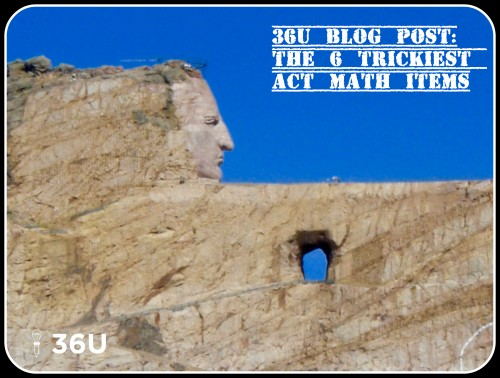The Six Trickiest Math Problems We Found on the Most Recent ACT

If you learned what your teachers taught in your math classes, then you should do great on the ACT math test. However, like most standardized tests, the ACT will throw a few items your way that look a little different than the items your teacher assigned.

So, here are the tricky items we found on the latest publicly-released ACT. There are six of them. Have questions or comments? Let us know! Please note: these aren’t necessarily the most difficult items on the math test, though some are, but they are the ones that we consider the slyest.

All items shown below are from the 2016-2017 Preparing-for-the-ACT Guide. They are the property of ACT Inc., not 36 University.

Tricky ACT Math Item #6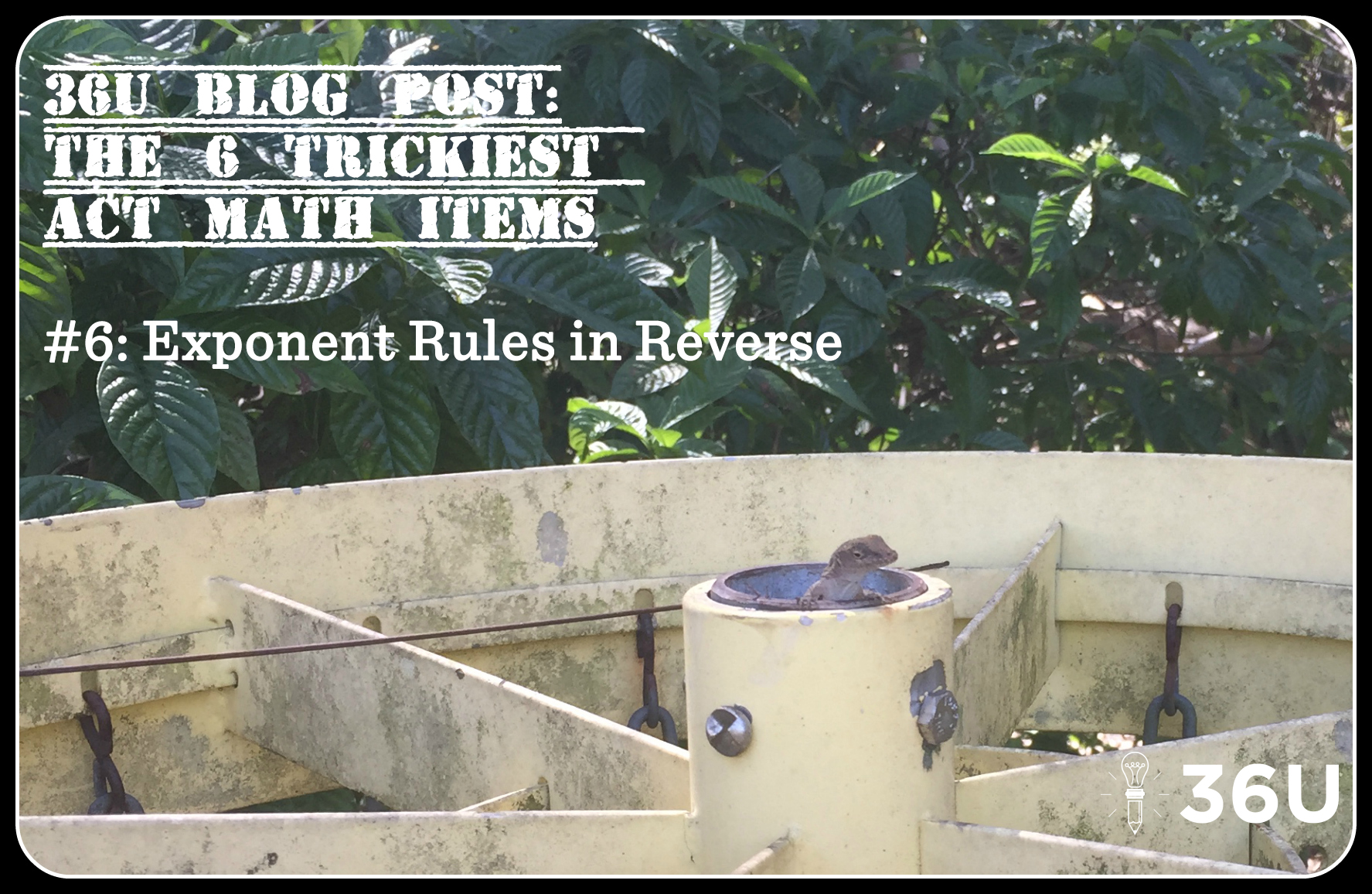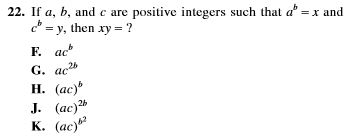This item is asking for an application of an exponents rule, but in a way that isn’t usually stressed in math classrooms. Usually, students are taught to distribute exponents to the bases:

(ab)x –> axbx

But this problem asks students to apply the same concept, but in reverse.

How You Should Work It

Step 1) Use the information from the problem (x = ab and y =cb) to substitute for x and y.

x•y –> abcb

Step 2) Apply the exponent rule (ab)x –> axbx in reverse. In other words, both bases have the same exponent, so the exponent can be placed outside parentheses:

abcb –> (ac)bTricky ACT Math Item #5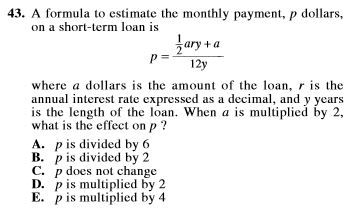Monthly payment. P dollars. Short-term loan. Annual interest rate. This item is wordy, and the vocabulary is a little tough. On top of that, that equation is intimidating.

On top of that, many students won’t know how to handle multiplying a by 2.

How You Should Work It

Step 1) Multiply a by 2.

Here’s the new value for p: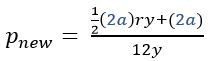Step 2) Factor out the 2.

Don’t make this too complicated: use the distributive property in reverse.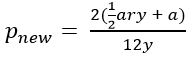and because multiplying the numerator by 2 is the same as multiplying the fraction by 2: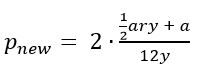Step 3) Compare to the previous value for p.

In the problem, the value for p was: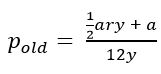When a is multiplied by 2, p is also multiplied by 2. The answer is D.Tricky ACT Math Item #4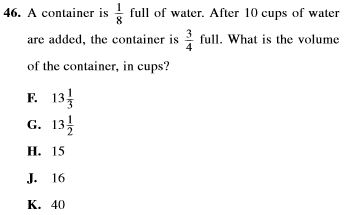Reasoning through this item, especially with its fractions, is very difficult. Only the top students may find that a navigable path.

How You Should Work It

The easiest way to work the item is to set up an equation that mirrors the situation.

Let x = volume of the container in cups

Step 1) Set up the equation.

The problem says they took 1/8 of the container, added 10 cups, and ended with the container 3/4 full. Or, maybe this is simpler: One-eighth of the container plus 10 cups equals three-fourths of the container.

As an equation, that description looks like this: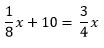Step 2) Solve the equation for x, the volume of the container.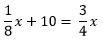Subtract (1/8)*x from both sides.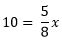Multiply both sides of the equation by 8/5.

x = 16 cups

It’s also a good practice to check your solution with the information given in the problem. Have fun!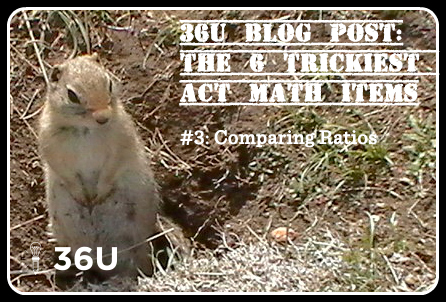Tricky ACT Math Item #3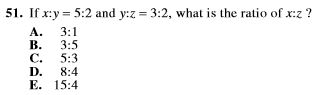Students are often more comfortable working from point A to point B along a path they’ve trod several times before. This item invites students to reason to try to find the relationship between x and z, but that reasoning is difficult.

How You Should Work It

There is a variable common in both ratios—y. Rewrite both ratios so that y has the same value in both.

Step 1) Rewrite both ratios.

The variable y corresponds with the 2 in the first ratio and a 3 in the second ratio. Rewrite both ratios so that y has a value of 6 (least common multiple).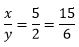and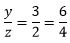Step 2) Make the comparison between x and z.

Rewriting both ratios as fractions with a y value of 6 allows us to compare x and z.

If the ratio of x to y is 15 to 6 and the ratio of y to z is 6 to 4, then the ratio of x to z is 15 to 4.

Tricky ACT Math Item #2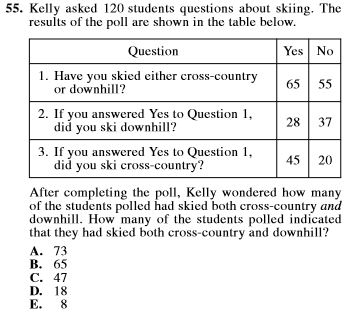This item, like a few others in this list, is likely to have students spending an inordinate amount of time with a trial-and-error method trying reason their way to the answer. That is a tough road!

How You Should Work It

Students need a Venn Diagram in their math tool chest. Unfortunately, many of them may not have used a Venn Diagram since the 8th grade.

Set up a Venn Diagram for all 120 students.

Step 1) Survey question one tells us that 55 students have neither skied cross-country or downhill.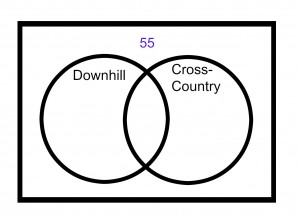Step 2) Use survey questions two and three to find the number of students who have skied both cross-country and downhill.

Survey question one states that 65 students have either skied cross-country or downhill. Survey question two states that 28 have skied downhill and 45 have skied cross-country. That’s 73 total, 8 more than the 65 from Q1. That means some of them have done both!

Take the surplus of 8 and place them in the overlap for students who have skied both downhill and country-country.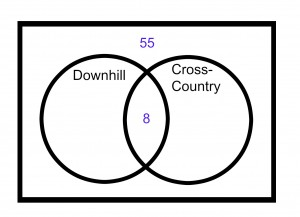Step 3) Use survey questions 2 and 3 to finish out the Venn Diagram.

Twenty-eight students have skied downhill.

Forty-five students have skied cross-country.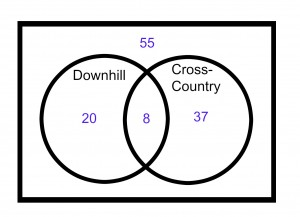Step 4) Check your Venn Diagram with the original information.

After your check, you’ll see that indeed 8 of the students had skied both cross-country and downhill.Tricky ACT Math Item #1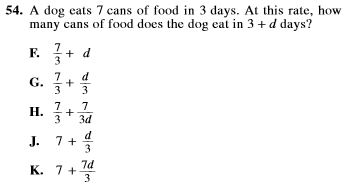There are two difficulties here:

1) Rate problems are standard fare in algebra class, but this item isn’t worked like most of those textbook problems are worked.

2) The wording is strange. Who ever asks about how many cans of food a dog eats in “’3 + d days?’”

How You Should Work It

Step 1) Recognize the rate at which the dog is eating.

Seven cans in three days means the dog is eating 7/3 of a can per day. This means that you can take the rate (7/3 of can per day) and multiply by the number of days to get the total amount of dog food consumed.

Try this: After 3 days the dog has eaten (7/3) * 3 = 7 cans of food, just like the problem stated.

Step 2) Substitute to find the solution.

How many cans does the dog eat in 3 + d days? Break this into two separate parts. In 3 days, the dog eats 7 cans, just like the problem told us. In d days, the dog eats (7/3)*d cans, just like we figured in Step 1.  This means the dog eats 7 + (7/3)*d cans.

Solution Page

The Locker ProblemWe want thank all who attempted the January 2017 Math Challenge. We hope you enjoyed and learned from the problem.

For those who missed it, here was the challenge problem:

Imagine 100 lockers numbered 1 to 100 with 100 students lined up in front of those 100 lockers:

The first student opens every locker.

The second student closes every 2nd locker.

The 3rd student changes every 3rd locker; if it’s closed, she opens it; if it’s open, she closes it.

The 4th student changes every fourth locker.

The 5th student changes every 5th locker.

That same pattern continues for all 100 students.

Here’s the question: “Which lockers are left open after all 100 students have walked the row of lockers?”

The Solution

As many of you found, the perfect square lockers (#s 1, 4, 9, 16, 25, 36, 49, 64, 81, and 100) are the only lockers left open. Cool, huh?

We hope you realized that lockers are only touched by students who are factors of that locker number, i.e. locker #5 is only touched by students 1 and 5. Student 1 opens it and student 5 closes it. In fact, because factors come in pairs, the first student factor will open it and the corresponding factor student closes it. At first, maybe you thought every locker would be closed because factors come in pairs. But there was a twist…

Here are a couple of ways you could have gotten there:

Method 1: Solve a Simpler Problem

We used a code: O = Open, C = Closed.

Here’s what the lockers look like after the first student walks through. They are all open.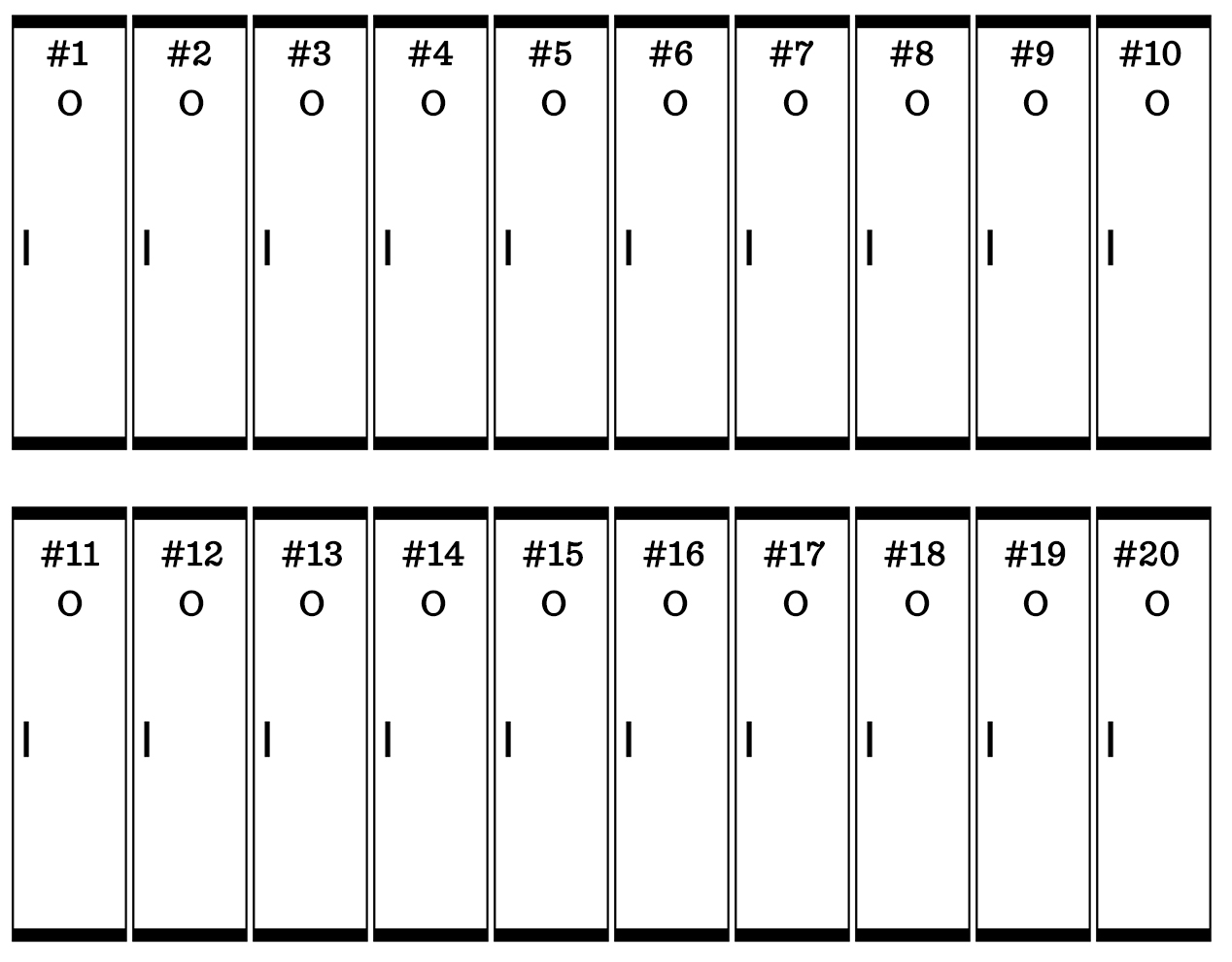After the second student walks the row of lockers, the odd-numbered lockers are left open and the even-numbered lockers are closed: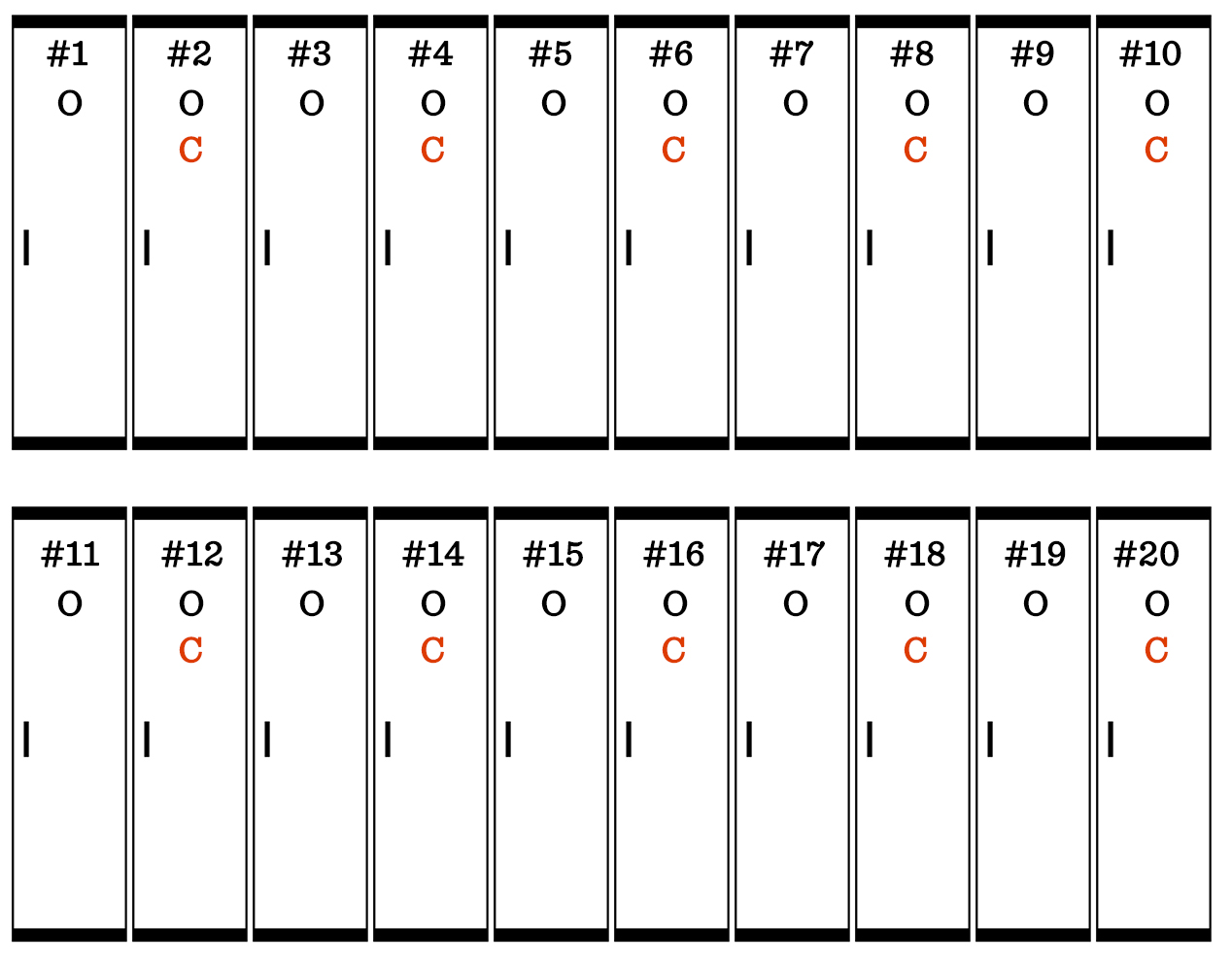Here’s how the lockers look after the third student changes every 3rd locker: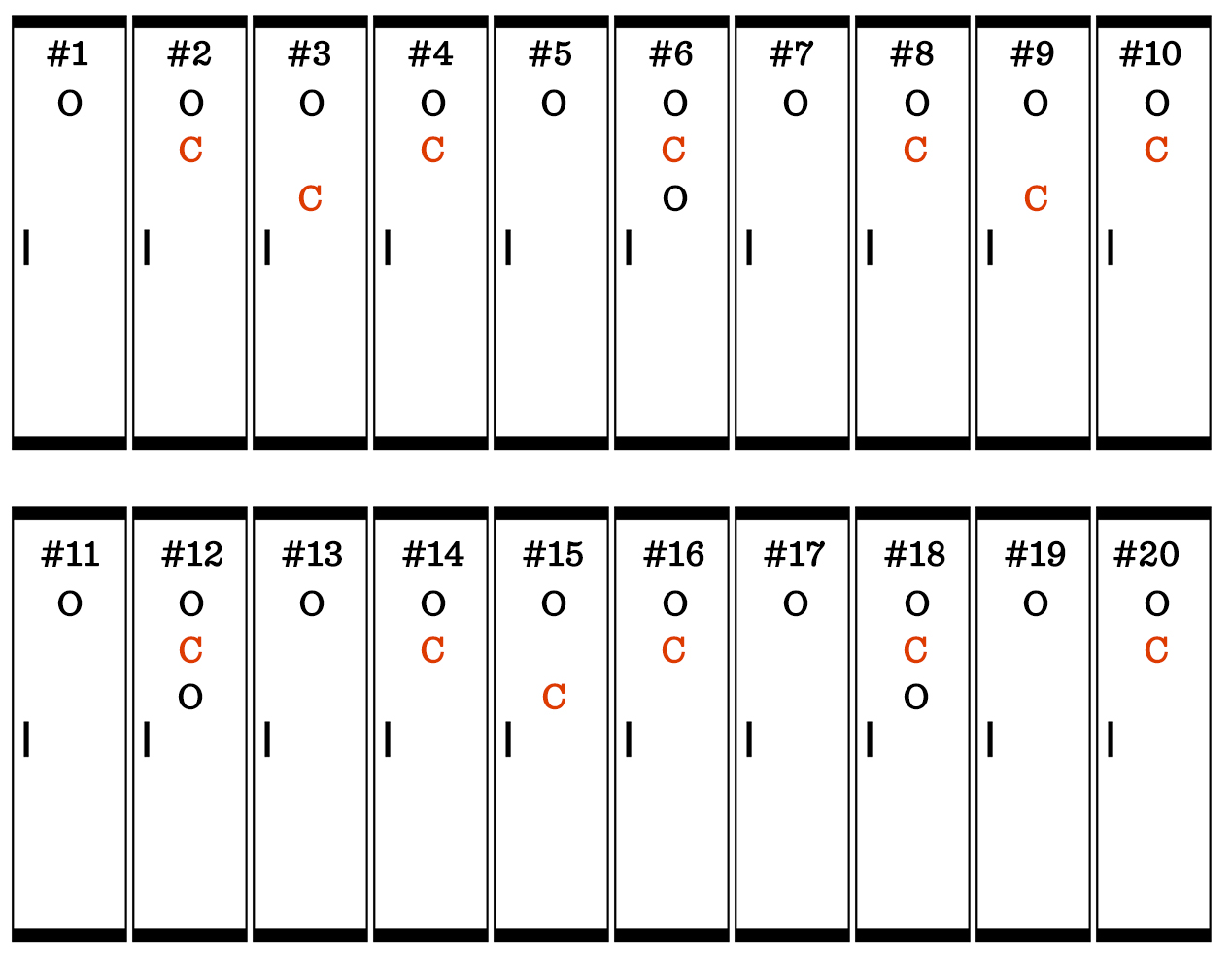And here’s how it looks after the first 20 students have walked the row of lockers. Note: after student 20 has gone, the first 20 lockers aren’t touched again.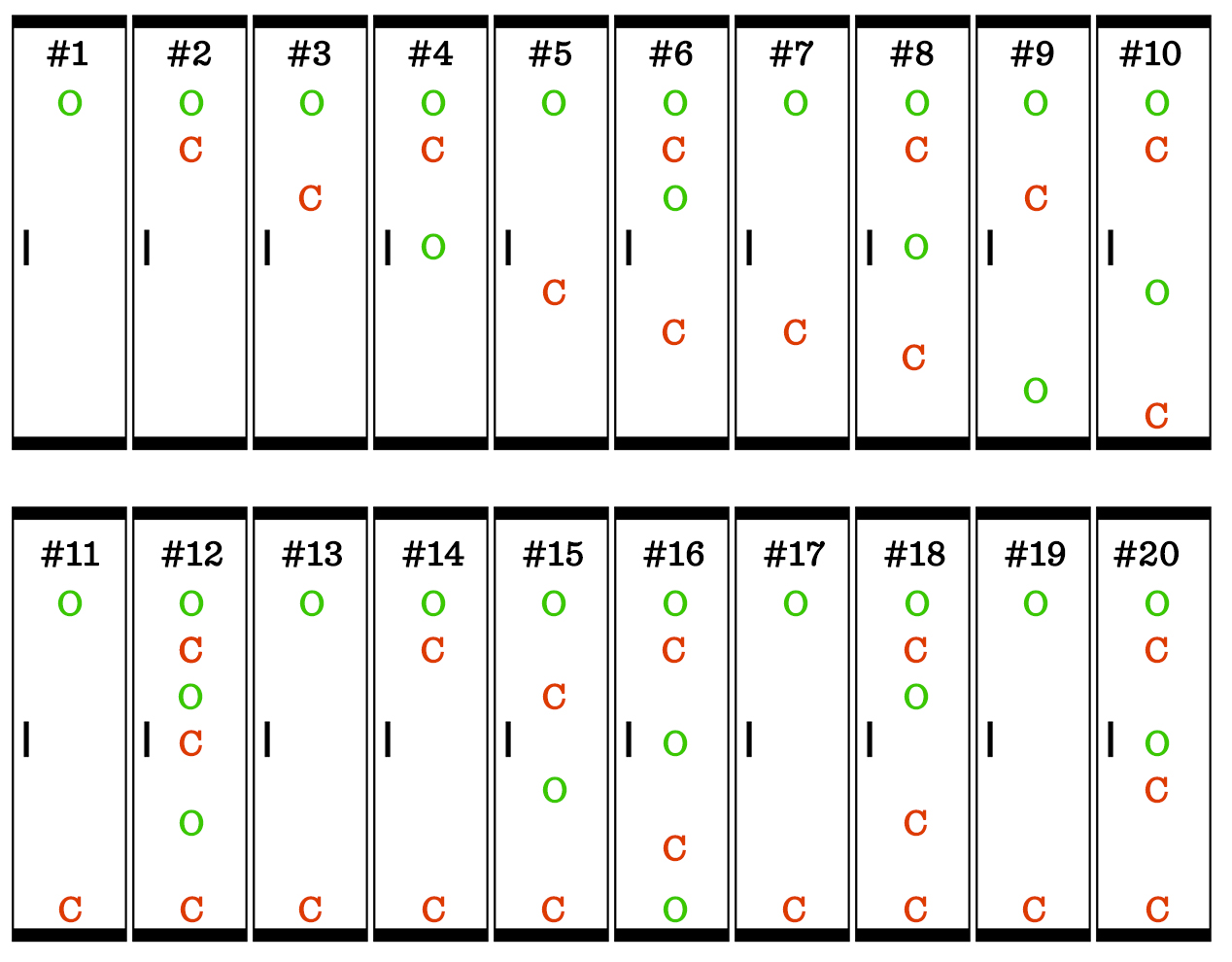Of the first 20 lockers, locker #s 1, 4, 9, and 16 are left open. Those are perfect squares. You can extend that pattern to identify the remaining open lockers.

Lockers 1, 4, 9, 16, 25, 36, 49, 64, 81, and 100 are left open!

Method 2: Who Touches Which Lockers

Identifying which students touch which lockers is a little less of a brute-force approach and would likely have gotten you to the solution a little more quickly.

Here’s what I mean:

Consider locker #1. The only student who touches locker #1 is student #1. Student 1 opens the locker, and since no one else touches it, it will be left open at the end.

Consider locker #2. Student 1 opens the locker, and student 2 closes it. No one else touches the locker, so it will be closed.

Consider locker #10. Students 1 opens the locker. Student 2 closes it. Students 3 and 4 skip right by it. Student 5 opens it. Students 6, 7, 8, and 9 skip right by it. And student 10 closes it. Locker #10 will be closed.

Mental Milestone 1: After looking at several lockers, you should notice that lockers are only changed by student numbers that are factors of the locker number. In other words, locker 12 is changed by students 1, 2, 3, 4, 6, and 12.

Mental Milestone 2: You should also have noticed that factors always come in pairs. This means that for every student who opens a locker, there is another student who closes it. For locker #12, student 1 opens it, but student 12 closes it later. Student 2 opens it, but student 6 closes it later. Student 3 opens it, but student 4 closes it later.

By this logic, every locker would be closed.

But there are exceptions!

Consider locker #25. Student 1 opens it. Student 5 closes it. Student 25 opens it. The locker will be left open, but why? In this case, the factors do not come in pairs. One and 25 are a pair, but five times five is also 25. Five only counts as one factor. This causes the open-close pattern to be thrown off. Locker #25 is left open.

Mental Milestone 3: When factors don’t come in pairs, the locker will be left open. And factors don’t come in pairs when numbers are multiplied by themselves. Perfect squares (1, 4, 9, 16, 25, 36, 49, 64, 81, 100,…) are the only numbers whose factors don’t come in pairs because one set of factors, the square root, is multiplied by itself. This means that only perfect square lockers will be left open.

Locker #s 1, 4, 9, 16, 25, 36, 49, 64, 81, and 100 are left open!

The Winner

Congratulations to Sydney H, our January 2017 ACT Challenge winner! Her solution was simple, precise, and just as important, correct. In fact, I like her solution better than the explanations I provided above. Here’s what she had to say: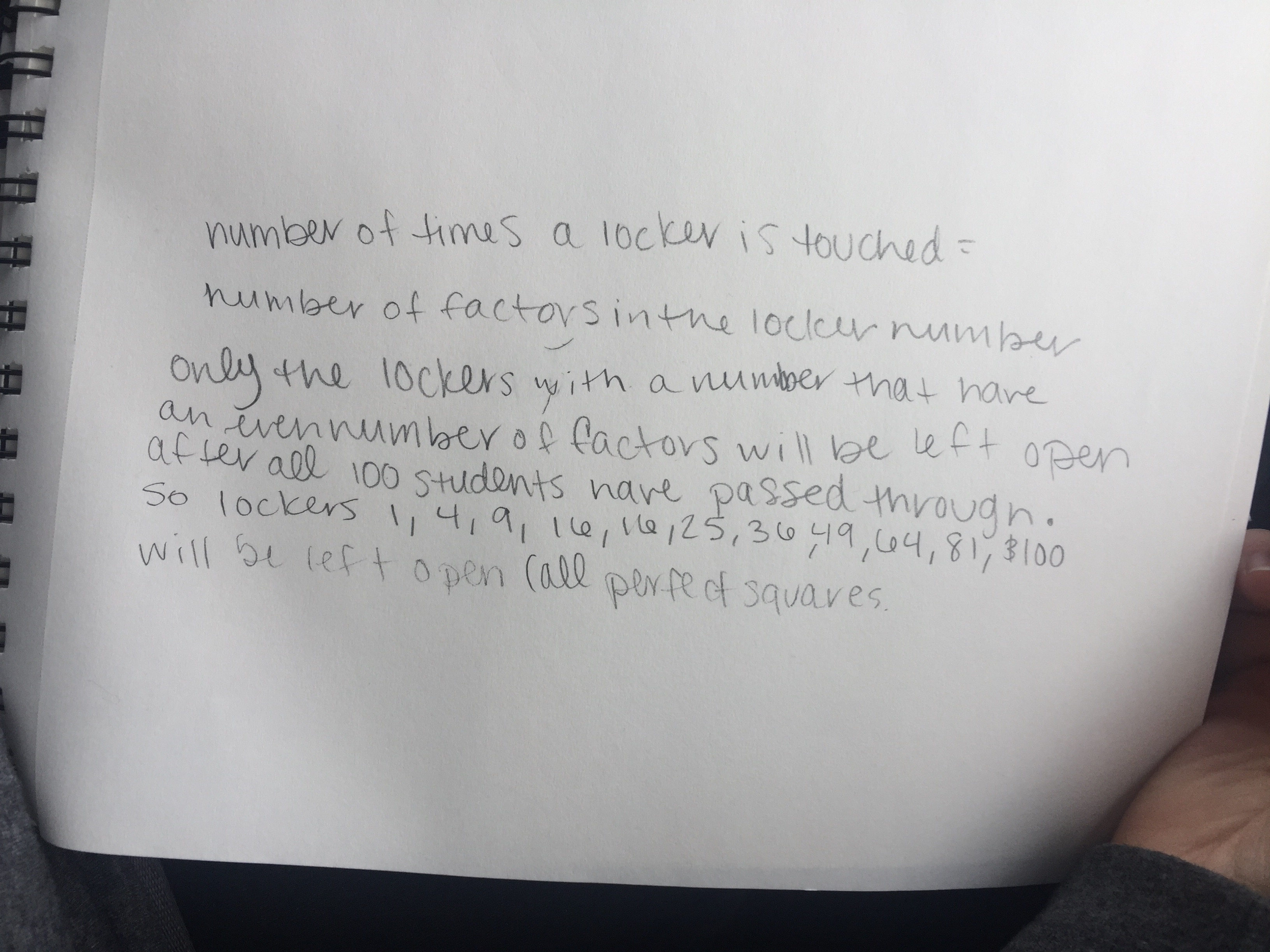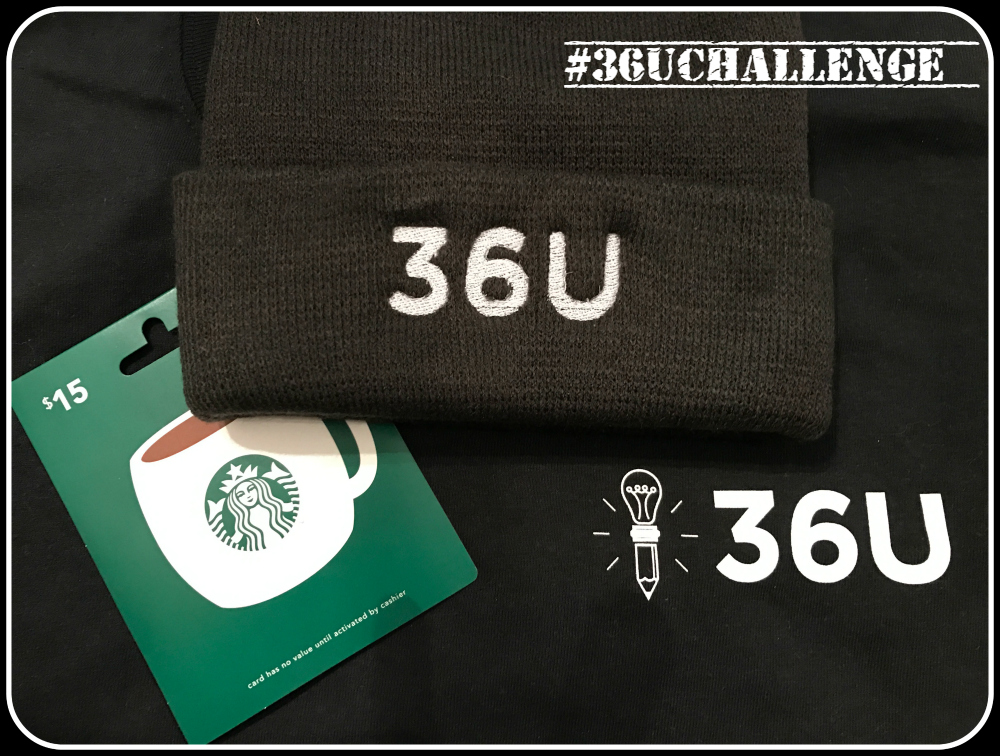The Winnings!

Sydney won the 36U Winter Care Package:

36U Tee (Long-sleeve)

36U toboggan

More 36U Resources:

36U Blog Posts

36U ACT Tips

36U ACT Prep Program

The Locker Problem – January 2017 ChallengeNumber properties are rarely reviewed, but they are sometimes tested on the ACT. So, we’ve decided to share a fun, straightforward, and hopefully enlightening item that will have you thinking about number properties. Here’s your January 2017 ACT Math Challenge:

Imagine 100 lockers numbered 1 to 100 with 100 students lined up in front of those 100 lockers:

The first student opens every locker.

The second student closes every 2nd locker.

The 3rd student changes every 3rd locker; if it’s closed, she opens it; if it’s open, she closes it.

The 4th student changes every fourth locker.

The 5th student changes every 5th locker.

That same pattern continues for all 100 students.

Here’s the question: “Which lockers are left open after all 100 students have walked the row of lockers?”

How Do I Enter?

Take a pic of your solution, with your work included, and post as a reply to any of our Locker Problem social media posts or send to lockerchallenge@36university.com. Submissions must be posted by midnight eastern time on January 31st. Impress us with your approach to solving this problem!

The winner will receive the 36U Winter Care Package:

36U Tee (Long-sleeve)

36U toboggan

How Will 36U Choose a Winner?

At 36U, we value simple, precise solutions. We will draw a winner on February 1st from among entries with correct answers and easy-to-understand explanations. (or: whose solutions are correct and whose approach is easily understood.)

More 36U Resources:

36U Blog Posts

36U ACT Tips

36U ACT Prep Program

Side-by-Side Comparison of the Updated ACT and the New SATWow! The SAT has changed. Instead of 10 smaller sections, now there are 4 bigger tests, just like the ACT.  The SAT no longer penalizes for guessing. And the essay is now optional. It has even begun incorporating science items in the English and reading tests. To top it off, question formatting is very similar, too. Let’s get right to it…

(This post contains screenshots of items released by ACT Inc. and the CollegeBoard (makers of the SAT). The items are not the property of 36 University.)

Test Order and Structure: ACT vs SAT

Both tests have 4 main tests and an optional essay.

 ACT SAT Section 1 English (75 questions in 45 min) Reading (52 questions in 65 min) Section 2 Math (60 questions in 60 min) Writing/Language (44 questions in 35 min) Section 3 Reading (40 questions in 35 min) Math (20 questions in 25 min). Last 5 are grid-in. Section 4 Science (40 questions in 35 min) Math (38 questions in 55 min). Last 8 are grid-in. Section 5 (Optional) Writing (Optional Essay in 40 min) Essay (Optional in 50 minutes) Total Time 2 hrs 55 min or 3 hrs 35 min w/ Essay 3 hrs or 3 hrs 50 min w/ Essay

Scoring

 ACT SAT Max Score 36 1600 Details Each test has max score of 36. Composite is average of four scores. SAT Reading max score: 800 SAT Reading derived from Reading & Writing/Language. SAT Math max score: 800 SAT Math score derived from 2 math sections. Composite is sum of Reading and Math scores.

There is no penalty for wrong answers on either test.

Writing scores are kept separate from the other scores on both tests.

Subject Comparisons

ACT English vs SAT Writing/Language

 ACT SAT Setup 75 questions in 45 minutes 44 questions in 35 minutes Pace 36 seconds per item ~48 seconds per item Question Format Both require you to evaluate underlined parts of passage.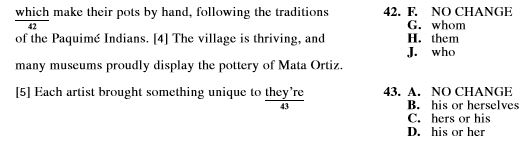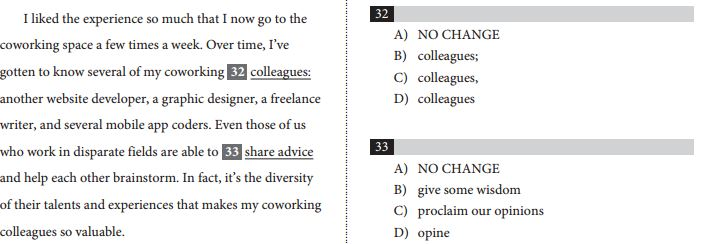Similar Question Type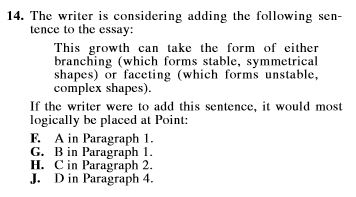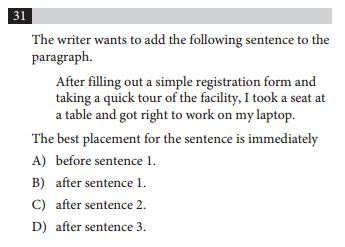Similar Question Type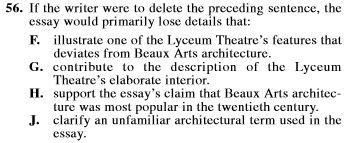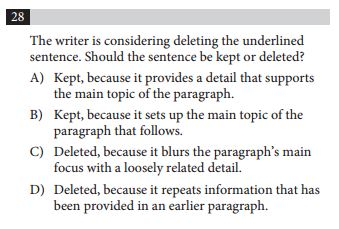Notes SAT English includes graphs.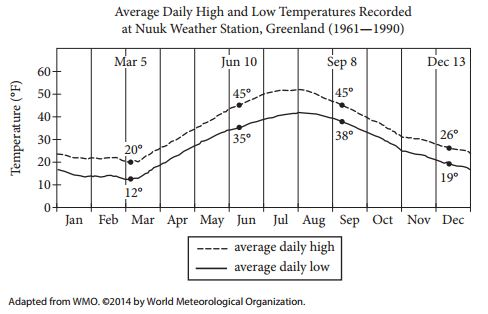The English tests on both the ACT and SAT have a very similar structure. The SAT English incorporates science graphs occasionally and seems to require a slightly more extensive vocabulary.

ACT Math vs SAT Math

 ACT SAT Setup 60 questions in 60 minutes Part 1 (no calculator): 20 questions in 20 minutes (15 MC, 15 Free Response) Part 2 (calculator): 38 questions in 55 minutes (30 MC, 8 Free Response) Pace 60 seconds per item Part 1: 60 seconds per item Part 2: ~87 seconds per item Question Format ACT Math has 5 answer choices. SAT Math has 4 answer choices on multiple choice questions. SAT Math also has some Grid-In answer items. Basic Items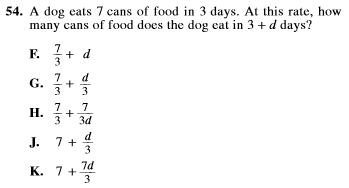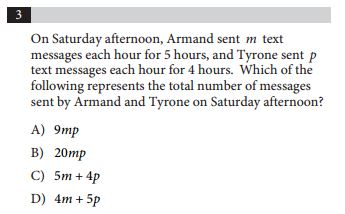Same Writers?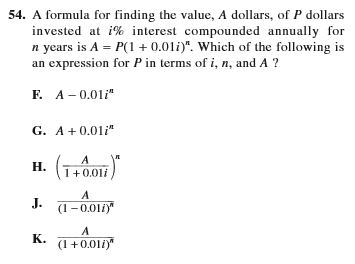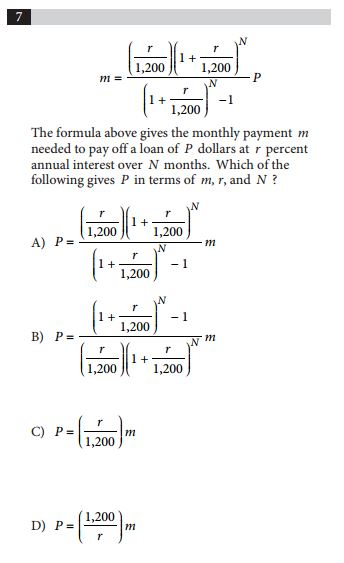Grid-Ins Not applicable.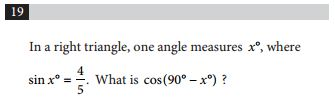***Students grid-in answers on answer document. Notes Calculator is allowed on all items. Calculator is allowed on Part 2, not Part 1.

The math tests are not as similar as the English tests.

 ACT SAT Setup 40 questions divided over 4 passages 35 minutes 52 questions divided over 5 passages 65 minutes Pace 52.5 seconds per item 75 seconds per item Passages 4 passages: Prose Fiction Social Science Humanities Natural Science 5 passages: U.S. or World Lit U.S. Constitution or Global Conversation Social Science 2 Science passages Comparison Items #1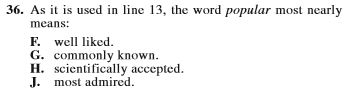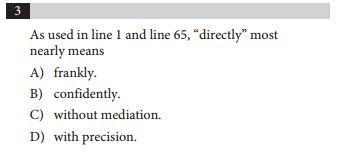Comparison Items #2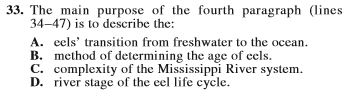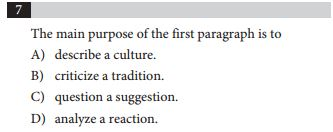Notes The SAT has begun adding items that require students to locate justification in the text.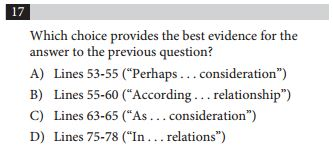Notes SAT Reading has science graphs included in the 2 science passages.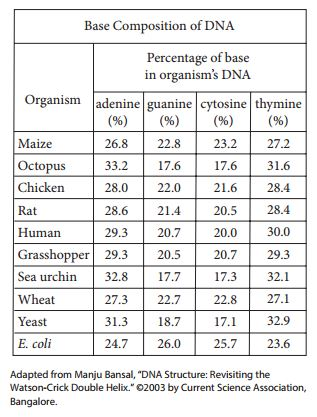As with the English tests, the Reading tests are very similar. On the SAT English tests, students will work with 2 science passages. On the ACT, the science is in a section on its own.

ACT Science in the SAT Writing and Reading

 ACT SAT Setup 40 questions divided over 6 or 7 scenarios 35 minutes N/A Pace 52.5 seconds per item N/A Question Format The ACT Science test requires students to interpret information in tables and graphs.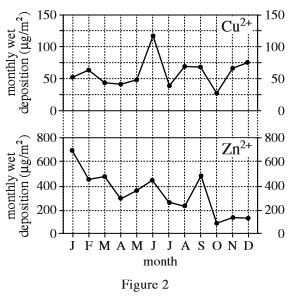The tables and graphs are incorporated in the SAT English and Reading tests. Basic Items Questions require students to evaluate experiments and research.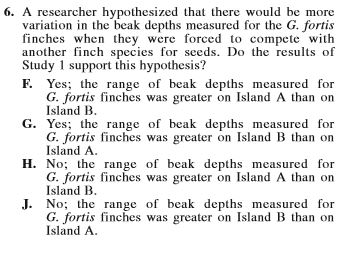The tables and graphs are incorporated in the SAT English and Reading tests.

ACT Writing vs SAT Essay

 ACT SAT Setup 1 essay in 40 minutes 1 essay in 50 minutes Format 1 Central Question. 3 Perspectives Provided. Students are asked to: -state and develop their own position. -analyze and evaluate the 3 perspectives in light of their stated position. Students are given a relatively lengthy passage to evaluate. Instead of stating their own position, they are asked to dissect, analyze, and evaluate the passage. Examples You can find an example of an ACT Writing test prompt provided by ACT Inc here: Sample Prompt The Collegeboard has two sample prompts available on its website: Prompt 1 Prompt 2

Final Word

I don’t consider one test more difficult than the other, and the new SAT is new enough that I haven’t completed enough practice tests to make a complete assessment. At this point, maybe the most obvious difference between the tests is that 1/4 of the ACT score is derived from math items and 1/2 of an SAT score comes from math items.

Kendal Shipley, Ed.D.
6/3/2016

Recent and Related Posts

The Story of Lil Carl and the Arithmetic Series

Get a Copy of Your ACT and Fix the Mistakes

“Can you help me with test anxiety?” #30secondswiththeCEO

36U Blog List

36U ACT Tips

#30SecondswiththeCEO Videos List

36U ACT Prep Program